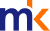Most Commented
Read more like thisNews
Bitcoin mining: Backbone of cryptocurrency, and its biggest problem

COMMENT | A few days after I submitted my previous article, the market capitalisation of cryptocurrencies plunged close to USD 423 billion on Dec 22, from an all-time high of slightly above USD 650 billion, just one day before – a whopping decline of 35 percent in 24 hours.

This came about as Emil Oldenburg, one of the founders of Bitcoin.org, publicly said that “an investment in Bitcoin right now I would say is the most risky investment you can make."He explained that increasing transaction fees, as well as the fundamental problem of the underlying mining process, could defeat the noble reason behind bitcoin’s existence in the first place - to be a peer-to-peer electronic cash system for the masses.

Here, I will explain the mining process, the way cryptocurrencies work, and the problem mining imposes on the way cryptocurrencies will work in the long term. Although the mechanisms of the mining process differ across cryptocurrencies, they are fundamentally similar.

Mining is the process of validating cryptocurrency transactions. In the bitcoin network, miners compete to validate a block of transactions, and creating a new block in the network. To “win” this competition, miners are required to solve complex cryptographic problems.

Simply put, imagine you are given two numbers – for instance, 2 and 50 – and you are required to tell the system the answer of the product of these numbers, which is unique. In this case, it would be 100.

Now, what if you are given the digit 100, and you are required to let the system know of the two unique numbers which lead to the digit earlier? In this case, we have 1;100, 2;50, 4;25, 5;20 and 10;10. This is simple enough, but as the problems get more complex, it takes a bit of a time to find the right answer.

We can make this problem more complicated by increasing the value of digit (e.g., in the billions), adding restrictions to the answer (e.g., determining that 1;100 and 100;1 are two different answers), and adding more mathematical problems to it (graphical solutions, prime number factors, indices, etc.).

Now, it will take longer for you to solve the mathematical problem. These are the fundamental building blocks of cryptography. Each problem contains the detail of the thousands of transactions, which is required to be validated and solved – which the answer, a SHA-256 hash, a string of 64 letters and numbers, will reflect...

Unlocking Article